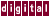When the `/scalaropt` level is 1 or higher, KAP performs dead-code elimination. The following optimizations are performed:

• Unreachable code removal

• Removal of zero-trip and empty `for` loops

• Elimination of resolved conditionals

• Removal of unprofitable code which requires `/scalaropt=2`

Each of the following code examples illustrates one of the optimizations:

1. Unreachable code removal, as shown in the following example:
``` goto hop;
x=2.0;
hop:
y=13.0;
```

Becomes:

```   hop:;
y = 13.0;
```

2. Removal of zero-trip and empty `for` loops. For example, the following would be deleted completely:
```    for (i=10;i<2;i++)
x = x+y;
```

3. Elimination of resolved conditionals, as shown in the following example:
```    #define NNN 12

if ( NNN > 10 )
x = 1.0;
else
x = 2.0;
```

Becomes:

```             x = 1.0;
```

The true branch will always be taken.

With the `/scalaropt` qualifier set to 2 and `/optimize` set > 2, the additional optimization explained in 4. is performed.

4. The removal of unprofitable code.

KAP performs lifetime analysis to determine the reaching definitions of variables and removes unused definitions, as shown in the following example:

``` y = 5.0;            /* no subsequent use */
x = 3.0;            /* variable redefined */
x = 4.0;
printf("%g \n",x);
```

Becomes:

``` x = 4.0;
printf("%g \n",x);
```

The full effect of dead-code elimination is realized when combined with other optimizations, such as subprogram inlining and forward substitution, which help in exposing useless or unreachable code.

Previous Page Next Page Contents Index
Command-Line Qualifiers